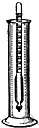# acidimetry

(redirected from acidimetrically)
Also found in: Thesaurus, Encyclopedia.

## ac·i·dim·e·ter

(ăs′ĭ-dĭm′ĭ-tər)
n.
A hydrometer used to determine the specific gravity of acid solutions.

ac′i·dim′e·try n.

## acidimetry

(ˌæsɪˈdɪmɪtrɪ)
n
(Chemistry) the determination of the amount of acid present in a solution, measured by an acidimeter or by volumetric analysis
 Noun 1acidimetry - volumetric analysis using standard solutions of acids to measure the amount of a base presentvolumetric analysis - quantitative analysis by the use of definite volumes of standard solutions or reagents